## ggplot2 in a nutshell

• Package for statistical graphics
• Designed to adhere to good graphical practices
• Supports a wide variety of plot types
• Constructs plots using the concept of layers
• For reference material refer to: http://had.co.nz/ggplot2/ or Wickham's book
• ggplot2: Elegant Graphics for Data Analysis

## qplot()

qplot() is the basic workhorse of ggplot2

• produces all plot types available with ggplot2
• allows for plotting options within the function statement
• creates an object that can be saved
• plot layers can be added to modify plot complexity

## qplot() structure

qplot() function has the following syntax:

qplot(variables, plot type, dataset, options)

• variables: list of variables used for the plot
• plot type: specified with a geom = statement
• dataset: specified with a data = statement
• options: there are so, so many options!

## NBA data

We will explore the NBA draft data set using ggplot2 for basic plotting. We will learning more advanced styling techniques is subsequent lessons.

nba <- read.csv("NBA Draft Class.csv")
head(nba)
##   Year Pick Team            Player Position
## 1 2008    1  CHI      Derrick Rose       PG
## 2 2008    2  MIA   Michael Beasley       SF
## 3 2008    3  MIN         O.J. Mayo       SG
## 4 2008    4  SEA Russell Westbrook       PG
## 5 2008    5  MEM        Kevin Love       PF
## 6 2008    6  NYK  Danilo Gallinari       SF
##                                 College Games Minutes Total.Points
## 1                 University of Memphis   289   10583         6017
## 2               Kansas State University   409   10170         5416
## 3     University of Southern California   435   14132         6447
## 4 University of California, Los Angeles   440   14932         8834
## 5 University of California, Los Angeles   364   11933         6989
## 6                            NoAttempts   285    8923         4138
##   Total.Rebounds Total.Assists Field.Goal.Percentage
## 1           1103          1954                 0.460
## 2           2007           539                 0.450
## 3           1414          1292                 0.433
## 4           2171          3045                 0.433
## 5           4453           898                 0.451
## 6           1327           546                 0.419
##   Three.Point.Percentage Free.Throw.Percentage Points.Per.Game
## 1                  0.312                 0.815            20.8
## 2                  0.348                 0.758            13.2
## 3                   0.38                 0.821            14.8
## 4                  0.305                 0.815            20.1
## 5                  0.362                 0.815            19.2
## 6                  0.369                 0.844            14.5
##   Rebounds.Per.Game Assists.Per.Game Win.Share     X
## 1               3.8              6.8      29.8 0.135
## 2               4.9              1.3      10.3 0.048
## 3               3.3              3.0      19.1 0.065
## 4               4.9              6.9      42.3 0.136
## 5              12.2              2.5      47.0 0.189
## 6               4.7              1.9      23.9 0.129

## Scatterplot

We first create a basic scatter plot of Win Share vs. Points Per Game. However, we must first load the ggplot2 library.

library(ggplot2)

Here we call qplot() with the following structure:

• Points.Per.Game: $$x$$-axis data points
• Win.Share: $$y$$-axis data points
• data = nba: tells us the data frame being used
• geom = "point": tells us that we are going to plot each point that is, create a scatter plot
• main = "…": denotes the title of our plot
qplot(Points.Per.Game,Win.Share, data = nba, geom = "point",
main = " Scatterplot of Win Shares vs. Points Per Game") # Title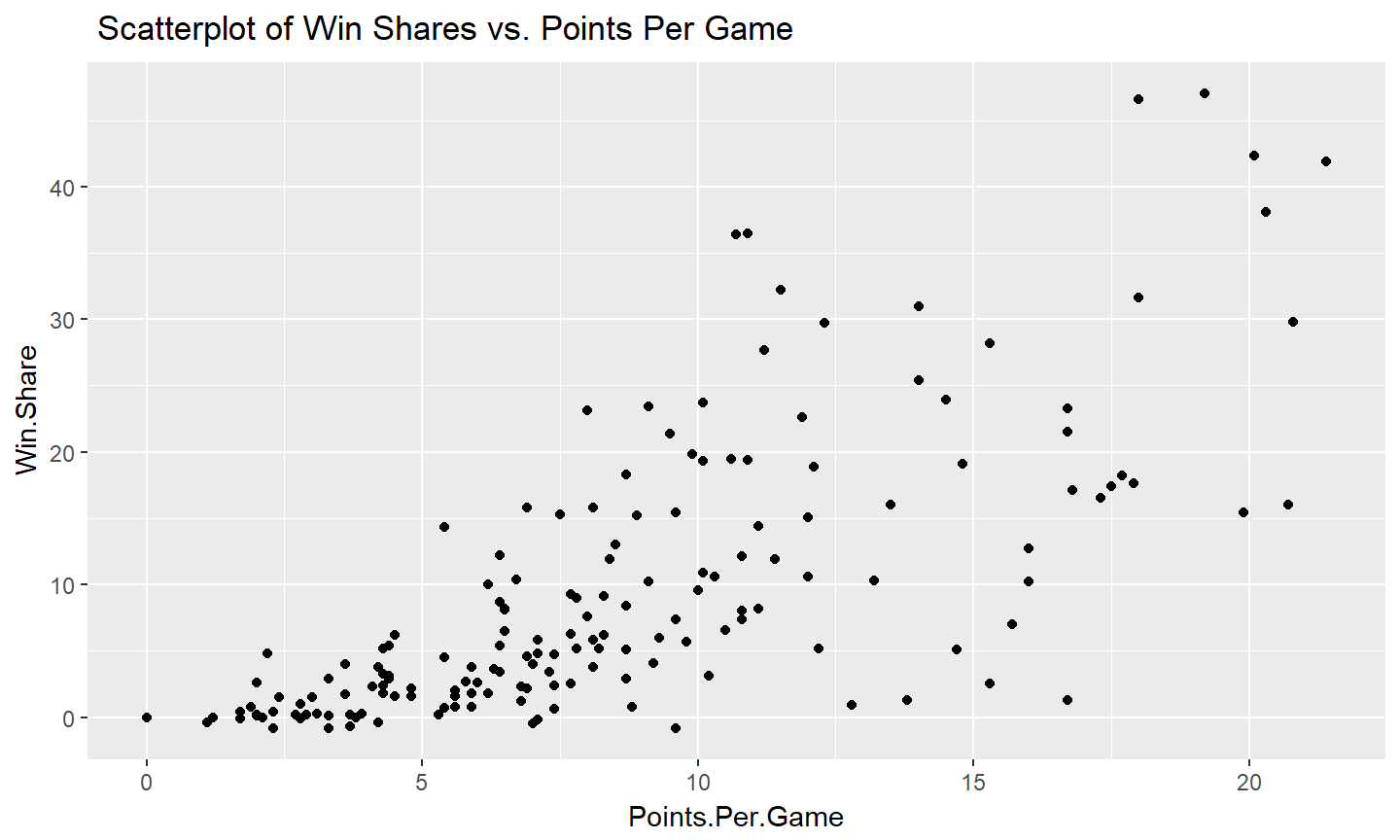## Scatterplot

Showing the versitility of options in qplot():

qplot(Points.Per.Game,Win.Share, data = nba, geom = "point",
colour = Position, # Assign colors based upon player position
main = "Win Share vs Points Per Game, Grouped by Player Position") # Title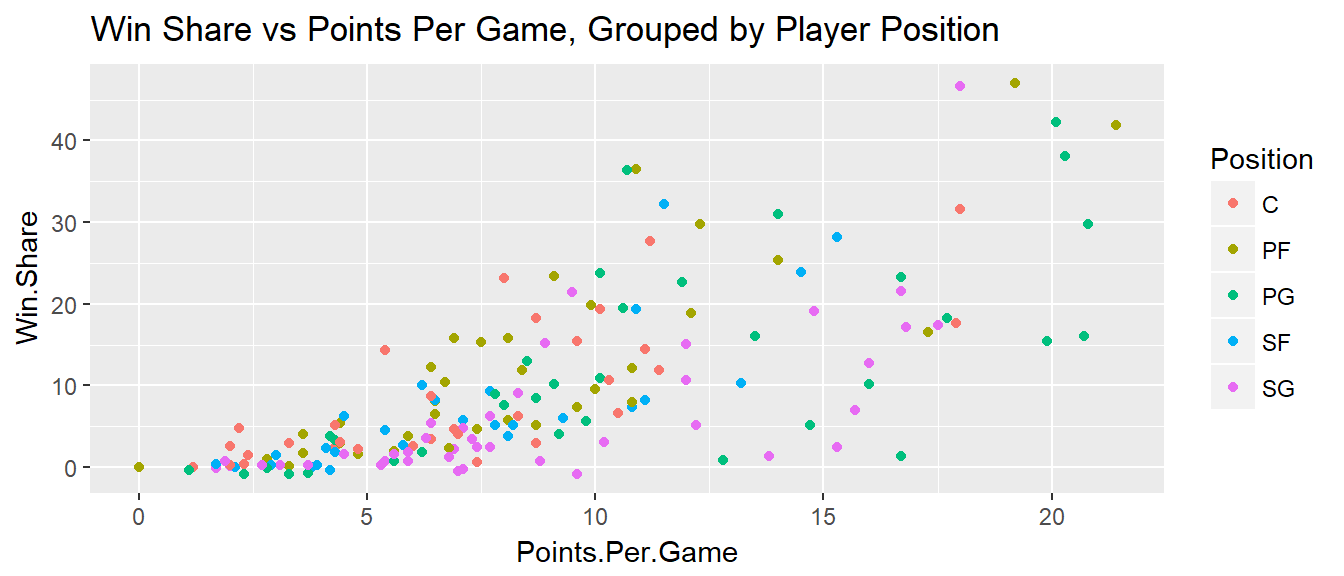To be explicit:

• colour = Position: parameter to color each point by its position
• main = "…": denotes the title of our plot

1. Create a scatterplot showing the relationship between Field Goal Percentage and Rebounds Per Game with Rebounds Per Game on the $$y$$-axis.
2. Use options within qplot() to color points by position.
3. Add a regression line using the geom_smooth(method = "lm",aes(group = 1)) command.

### 1.

qplot(Field.Goal.Percentage,Rebounds.Per.Game, data = nba, geom = "point",
main = "Rebounds Per Game vs. Field Goal Percentage")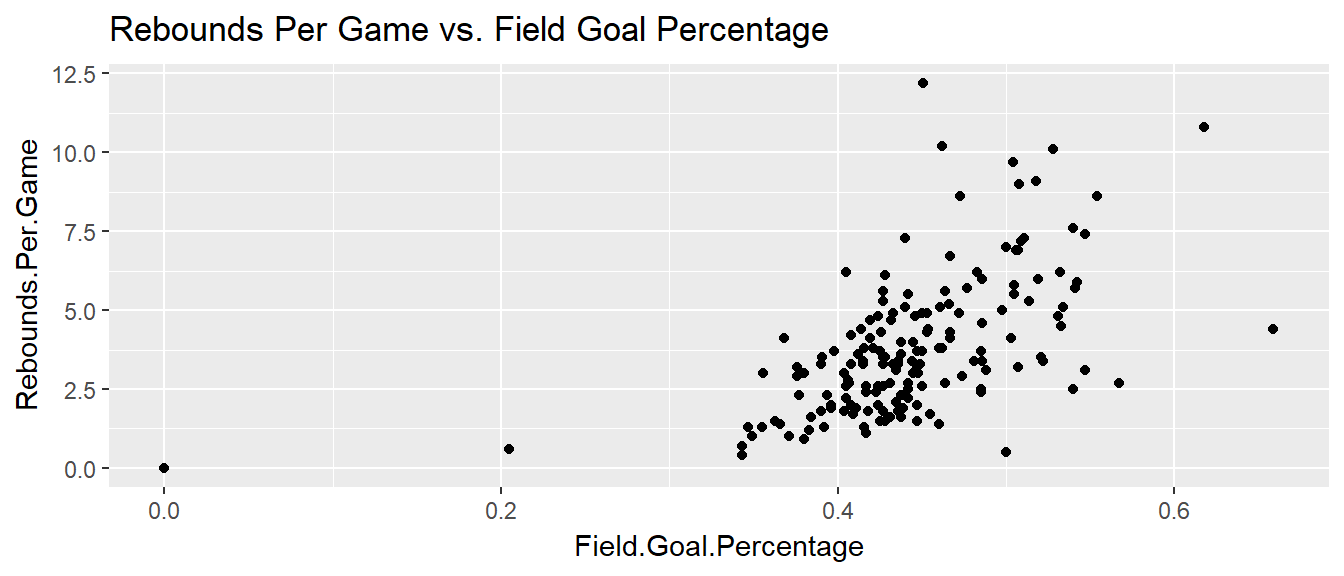### 2.

qplot(Field.Goal.Percentage,Rebounds.Per.Game, data = nba, geom = "point",
colour = Position, # Assign colors based upon player position
main = "Rebounds Per Game vs. Field Goal Percentage")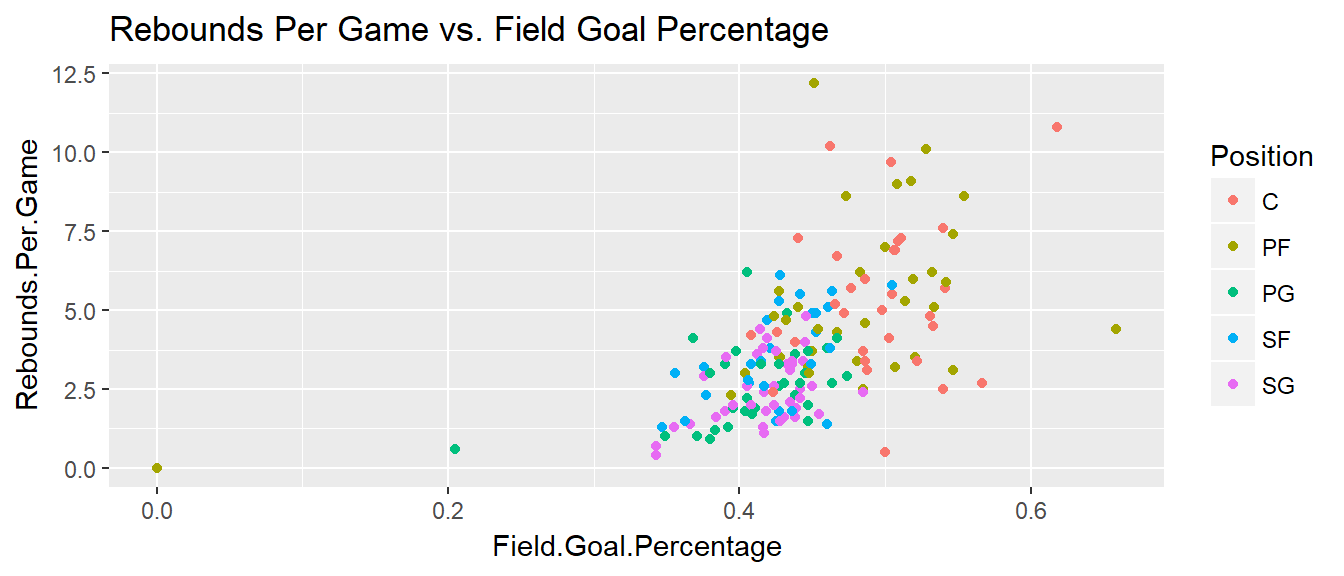### 3.

qplot(Field.Goal.Percentage,Rebounds.Per.Game, data = nba, geom = "point",
colour = Position, # Assign colors based upon player position
main = "Rebounds Per Game vs. Field Goal Percentage") +
geom_smooth(method = "lm",aes(group = 1))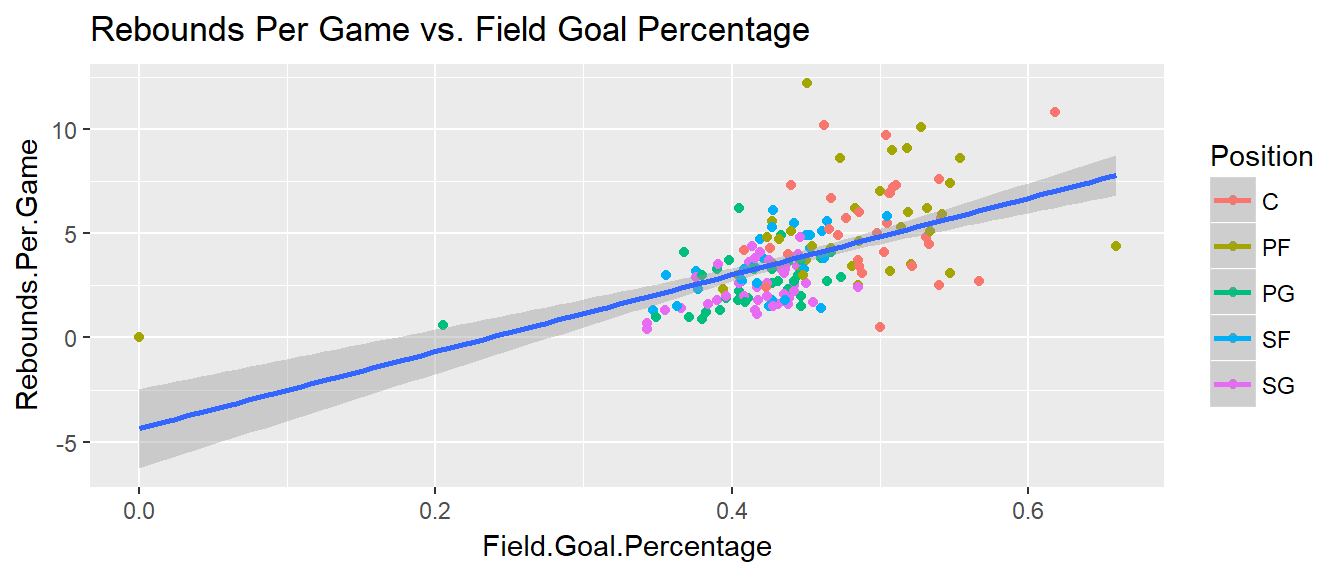## Histogram

Basic histogram of player positions

qplot(Points.Per.Game,data = nba, geom = "histogram",
fill = I("orange"), # Fills each bar orange
color = I("black"), # Outline bars in black
main = "Histogram of Points Per Game") # Title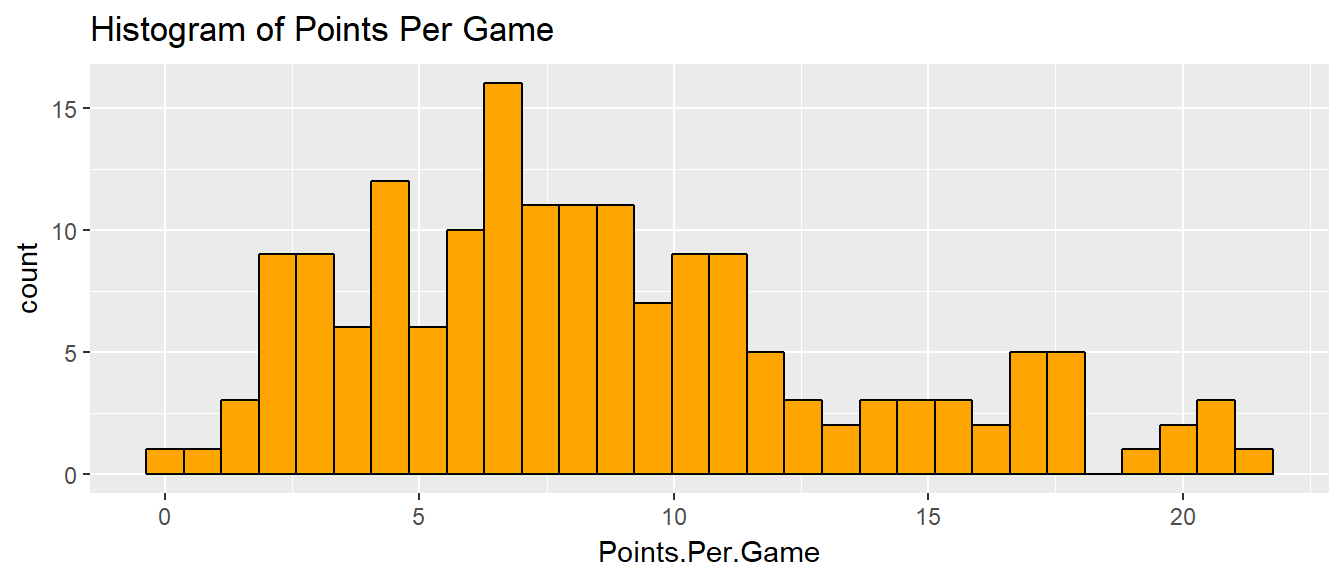## Histograms

Here we create a facet plot, that is a plot with multiple groups. In particular, our groups are the player positions.

qplot(Points.Per.Game,data = nba, geom = "histogram",
facets =.~Position,
binwidth = .6, # binwidth is the length of each rectangle
main = "Histogram of Points Per Game Faceted by Positions") # Title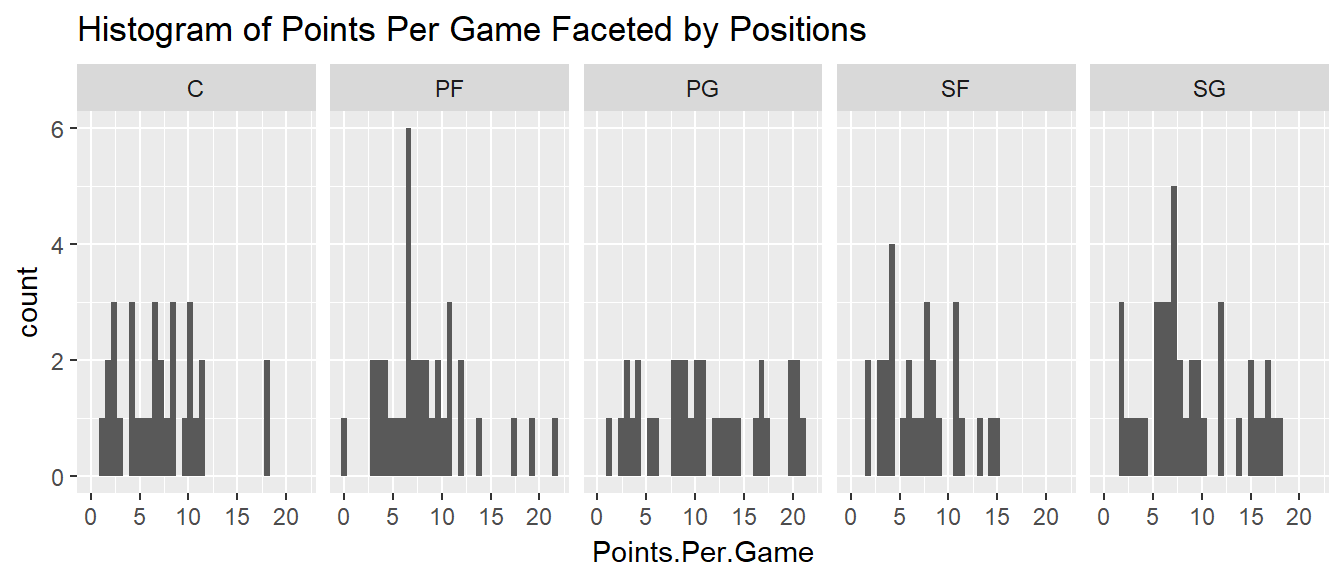1. Looking at a histogram, is there a position that seems to contribute least to win shares?
2. How does changing the bin width parameter affect the readability of the plot?

### 1.

qplot(Win.Share,data = nba, geom = "histogram",
facets =.~Position,
binwidth = 1, # binwidth is the length of each rectangle
main = "Histogram of Win Shares Faceted by Positions") # Title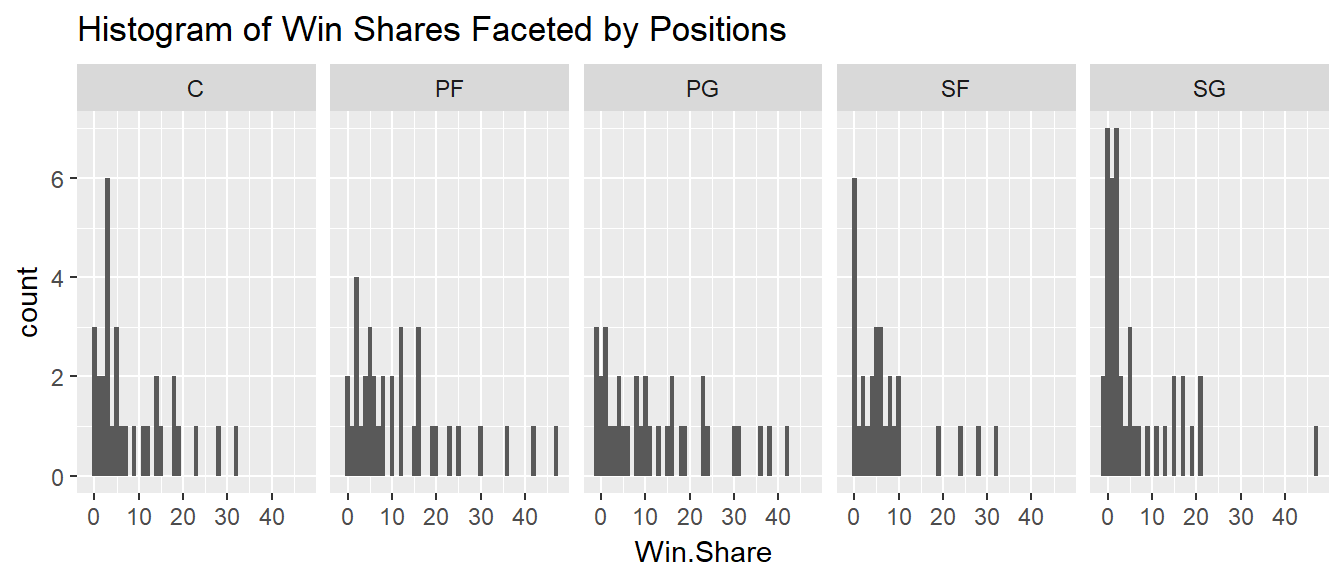More shooting guards had very low win shares than other positions.

### 2.

This is personal preference, but a small bin width is more readible for facetted plots.

## Boxplots

Side by side boxplot of points per game within each position:

qplot(Position,Points.Per.Game,data = nba, geom = "boxplot",
main = "Box Plot of Points Per Game by Position")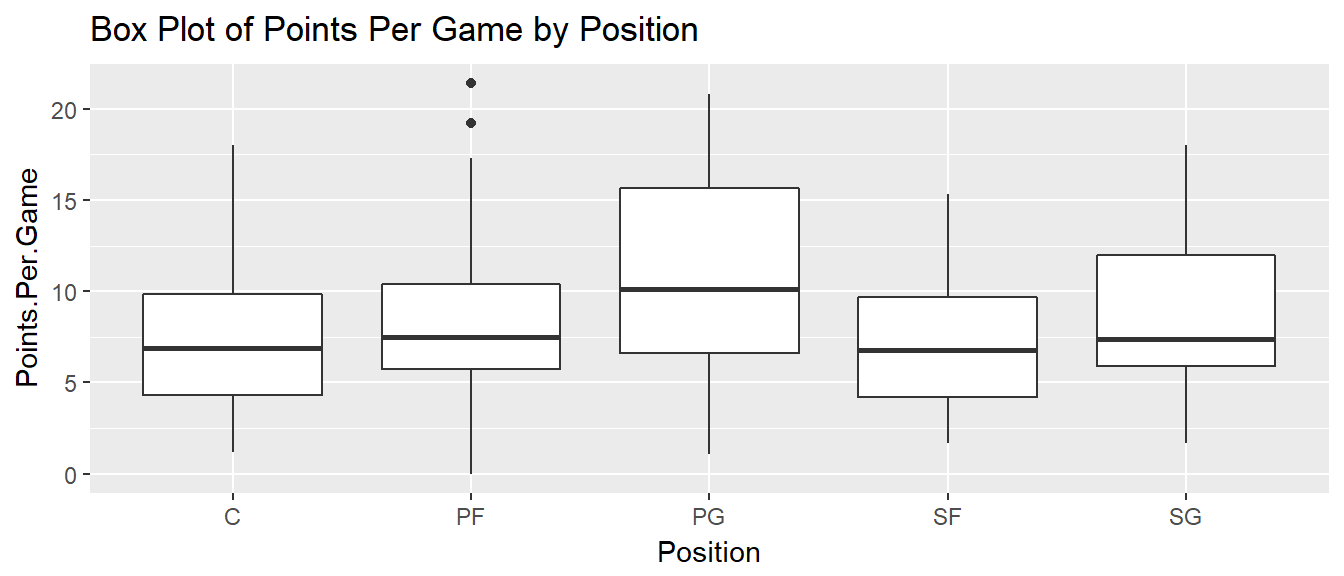## Boxplots

Side by side boxplot of log points per game within position groups with jittered values overlayed.

qplot(Position,log(Points.Per.Game),data = nba, geom = "boxplot",
main = "Box Plot of log Points Per Game Grouped by Position with Jittered Values") +
geom_jitter(alpha = I(.25))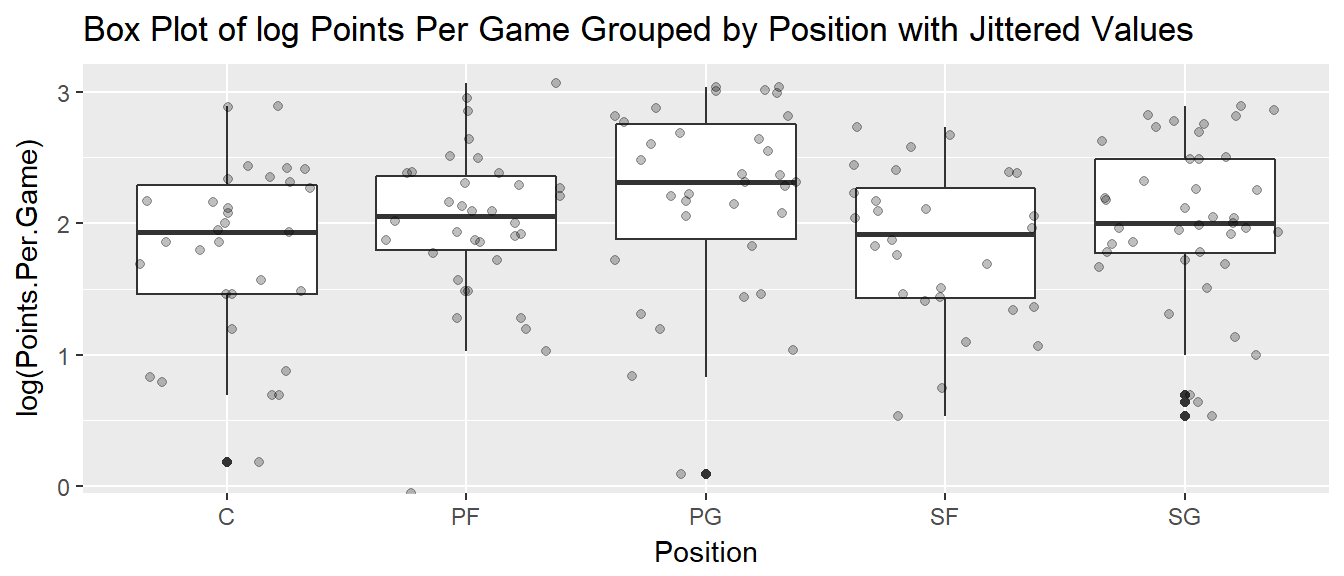1. Make side by side boxplots of win shares for each draft class. Use as.factor(Year) for the $$x$$-axis.
2. Overlay jittered points for observed values onto this boxplot.
3. Can we make a claim over which draft class is superior?

### 1.

qplot(as.factor(Year),Win.Share,data = nba, geom = "boxplot",
main = "Box Plot of win shares Grouped by Draft Class")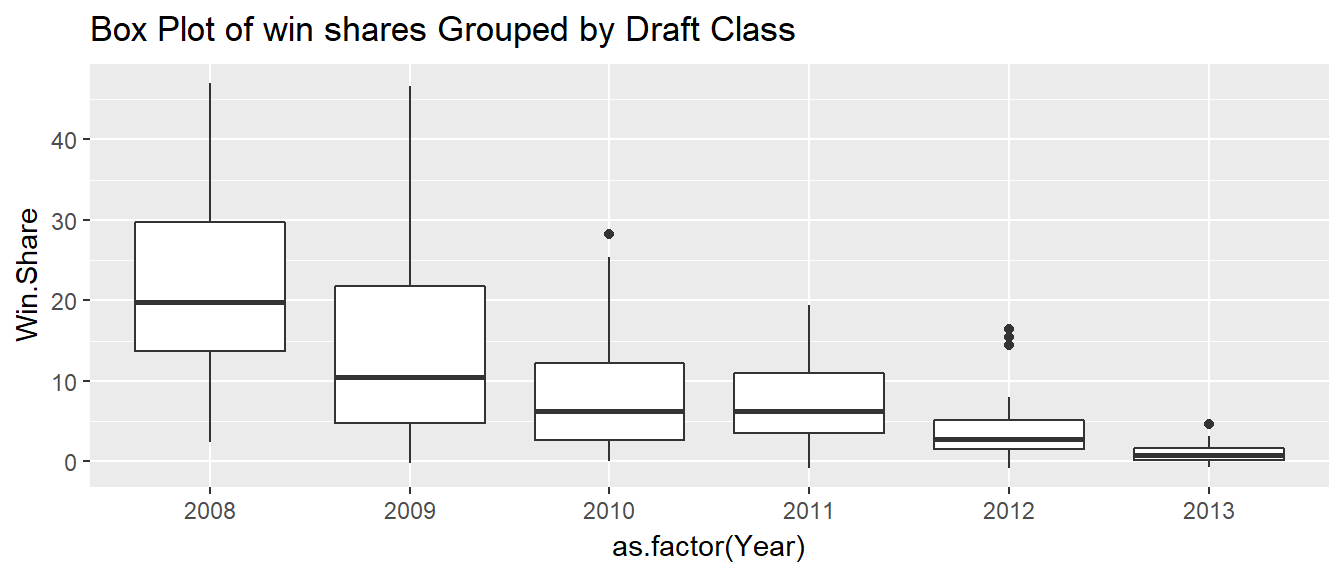### 2.

qplot(as.factor(Year),Win.Share,data = nba, geom = "boxplot",
main = "Box Plot of win shares Grouped by Draft Class") +
geom_jitter(alpha = I(.35))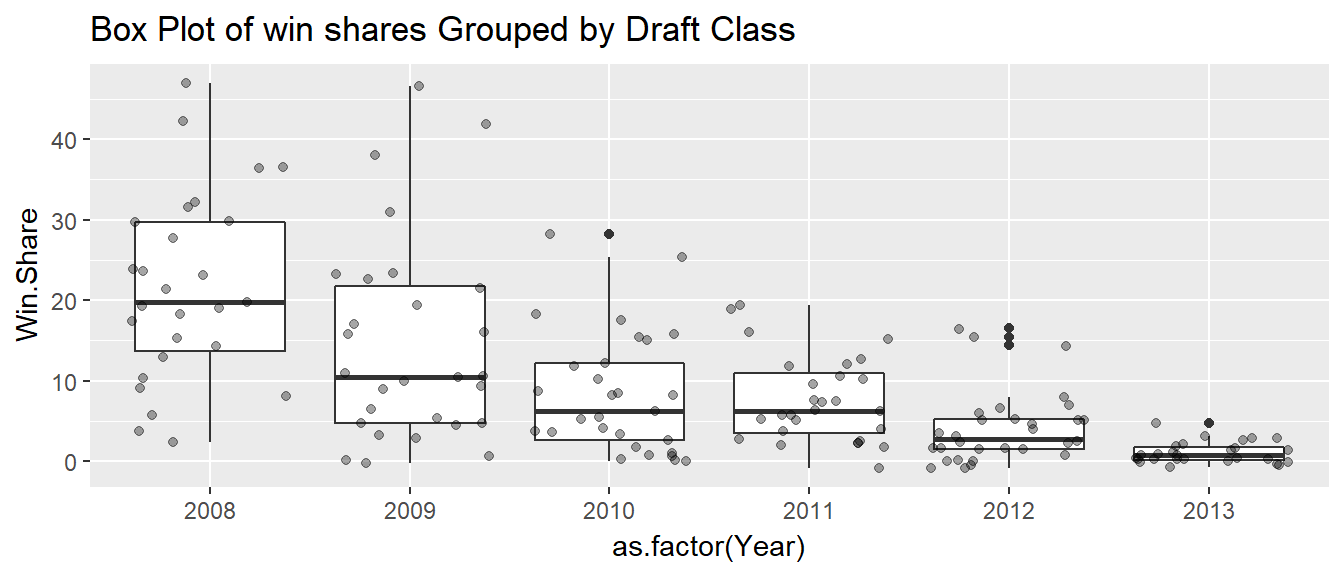### 3.

Comparing medians, 2008 has the highest win shares. However, this should be expected as those players have been in the league for 6 years, as opposed to the 2013 class that has only been in the NBA for 1 year. Using the data up until 2014, the class of 2008 is the most superior.

## Barplot

Barplot of different positions that were drafted in the first round from 2008-2013

qplot(Position,data = nba, geom = "bar",
main = "Bar Plot of Positions Drafted")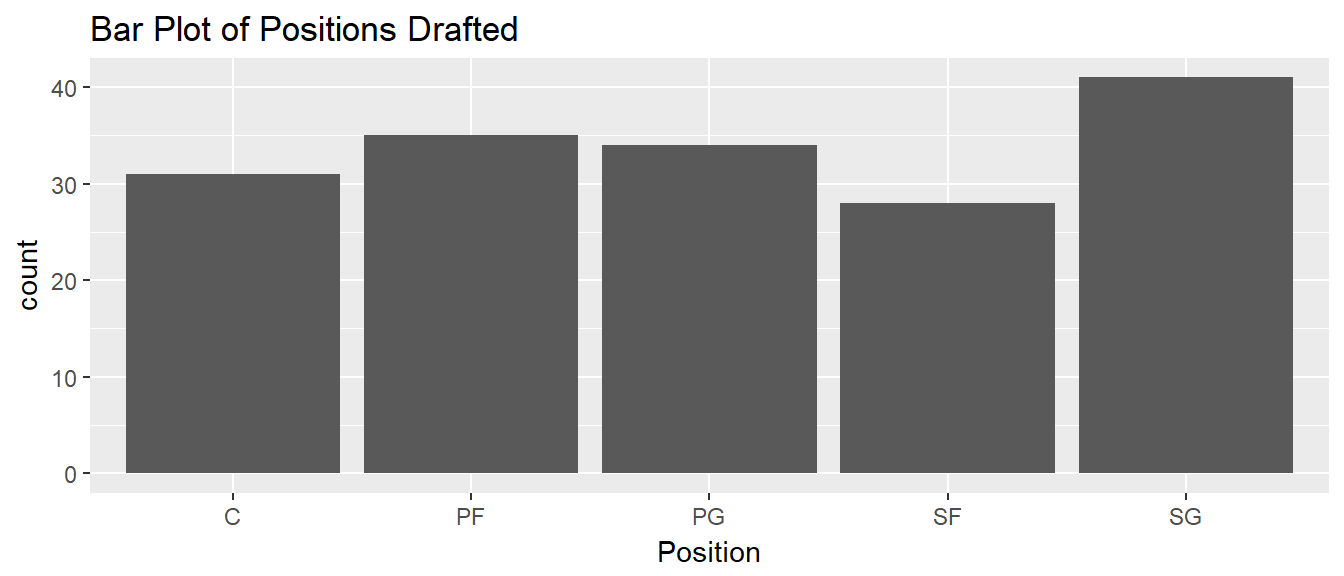## Barplot

Bar plot of different positions that were drafted in the first round from 2008-2013 faceted by years

qplot(Position,data = nba, geom = "bar",
facets = .~Year,
main = "Bar Plot of Positions Drafted Faceted by Position")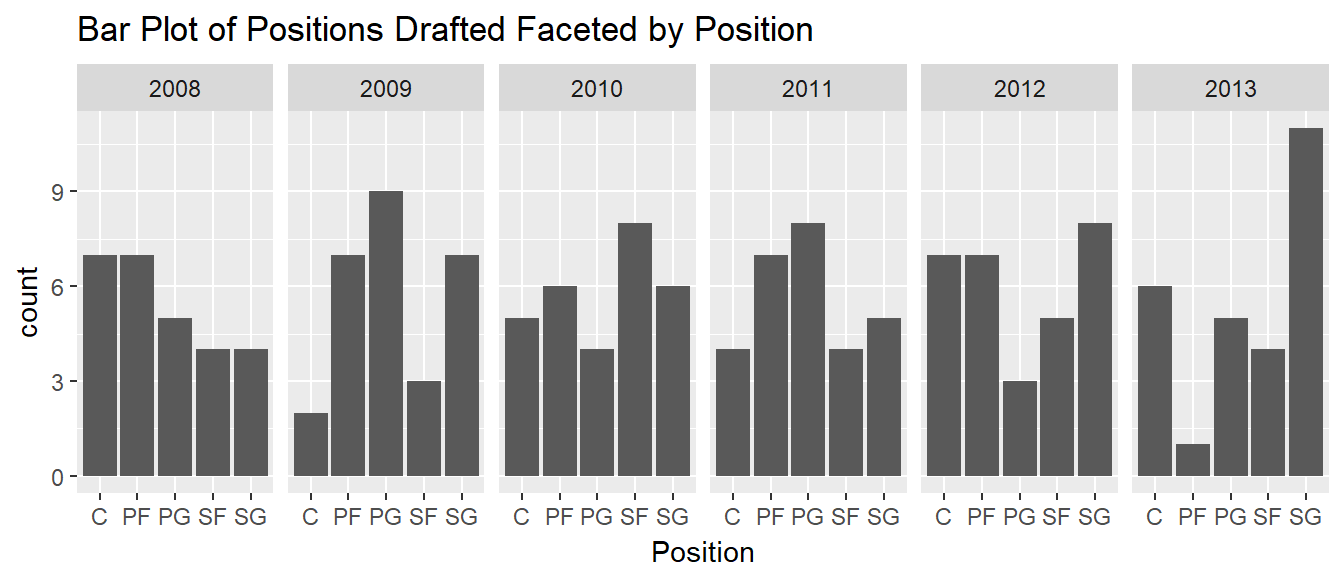1. Create a bar plot for the assists per game facet by position
2. Which position class seems to have the least assists per game?

Challenge: Did the University of Kentucky or University of Kansas provide more first round picks from 2008-2013? (Use any plotting method)

### 1.

qplot(Assists.Per.Game,data = nba, geom = "bar",
facets = .~Position,
main = "Bar Plot of Points Per Game Drafted Faceted by Position")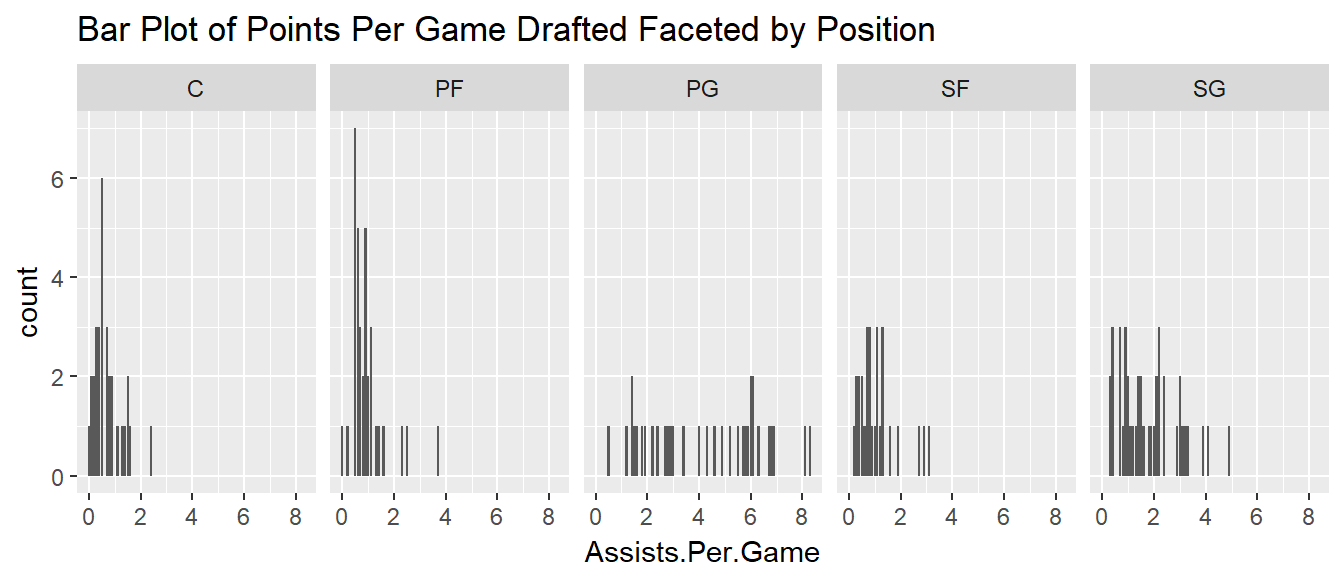### 2.

Centers seemly have the least amount of assists per game, and power forwards come in a close second.

### Challenge

qplot(College,
# Line below subsets the data frame
data = nba[nba\$College %in% c("University of Kansas","University of Kentucky"),],
geom = "bar",
main = "Number of Draft Picks from University of Kansas vs. University of Kentucky")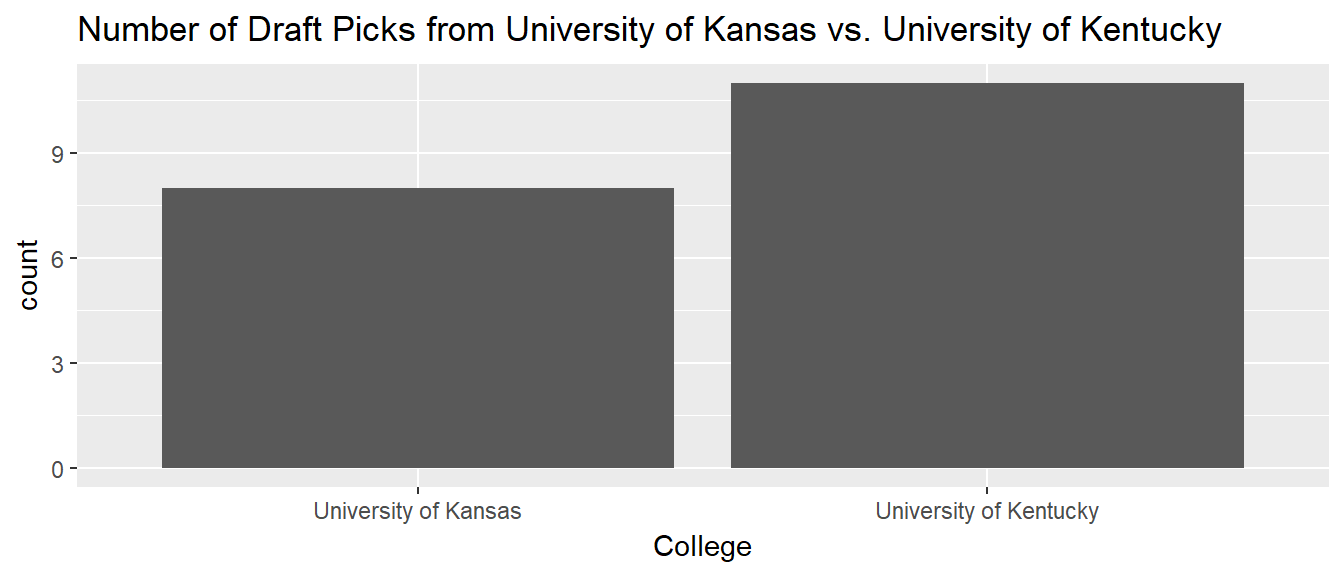The University of Kentucky had more draft picks than the University of Kansas.